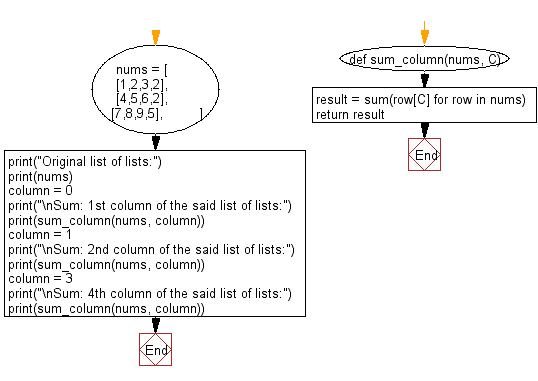﻿ Python: Sum a specific column of a list in a given list of lists - w3resource# Python: Sum a specific column of a list in a given list of lists

## Python List: Exercise - 142 with Solution

Write a Python program to sum a specific column of a list in a given list of lists.

Sample Solution:

Python Code:

``````def sum_column(nums, C):
result = sum(row[C] for row in nums)
return result

nums = [
[1,2,3,2],
[4,5,6,2],
[7,8,9,5],
]
print("Original list of lists:")
print(nums)

column = 0
print("\nSum: 1st column of the said list of lists:")
print(sum_column(nums, column))
column = 1
print("\nSum: 2nd column of the said list of lists:")
print(sum_column(nums, column))
column = 3
print("\nSum: 4th column of the said list of lists:")
print(sum_column(nums, column))
```
```

Sample Output:

```Original list of lists:
[[1, 2, 3, 2], [4, 5, 6, 2], [7, 8, 9, 5]]

Sum: 1st column of the said list of lists:
12

Sum: 2nd column of the said list of lists:
15

Sum: 4th column of the said list of lists:
9
```

Flowchart:## Visualize Python code execution:

The following tool visualize what the computer is doing step-by-step as it executes the said program:

Python Code Editor:

Have another way to solve this solution? Contribute your code (and comments) through Disqus.

What is the difficulty level of this exercise?

Test your Python skills with w3resource's quiz

﻿

## Python: Tips of the Day

Floor Division:

When we speak of division we normally mean (/) float division operator, this will give a precise result in float format with decimals.

For a rounded integer result there is (//) floor division operator in Python. Floor division will only give integer results that are round numbers.

```print(1000 // 300)
print(1000 / 300)```

Output:

```3
3.3333333333333335```